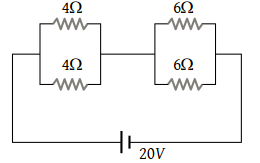# NEET Questions Solved

Four resistances are connected in a circuit in the given figure. The electric current flowing through 4 ohm and 6 ohm resistance is respectively(1) 2 amp and 4 amp

(2) 1 amp and 2 amp

(3) 1 amp and 1 amp

(4) 2 amp and 2 amp

(4) Equivalent resistance $=\frac{4×4}{4+4}+\frac{6×6}{6+6}=5ohm$

So the current in the circuit $=\frac{20}{5}=4$ampere

Hence the current flowing through each resistance = 2 ampere.

Difficulty Level:

• 10%
• 12%
• 14%
• 66%